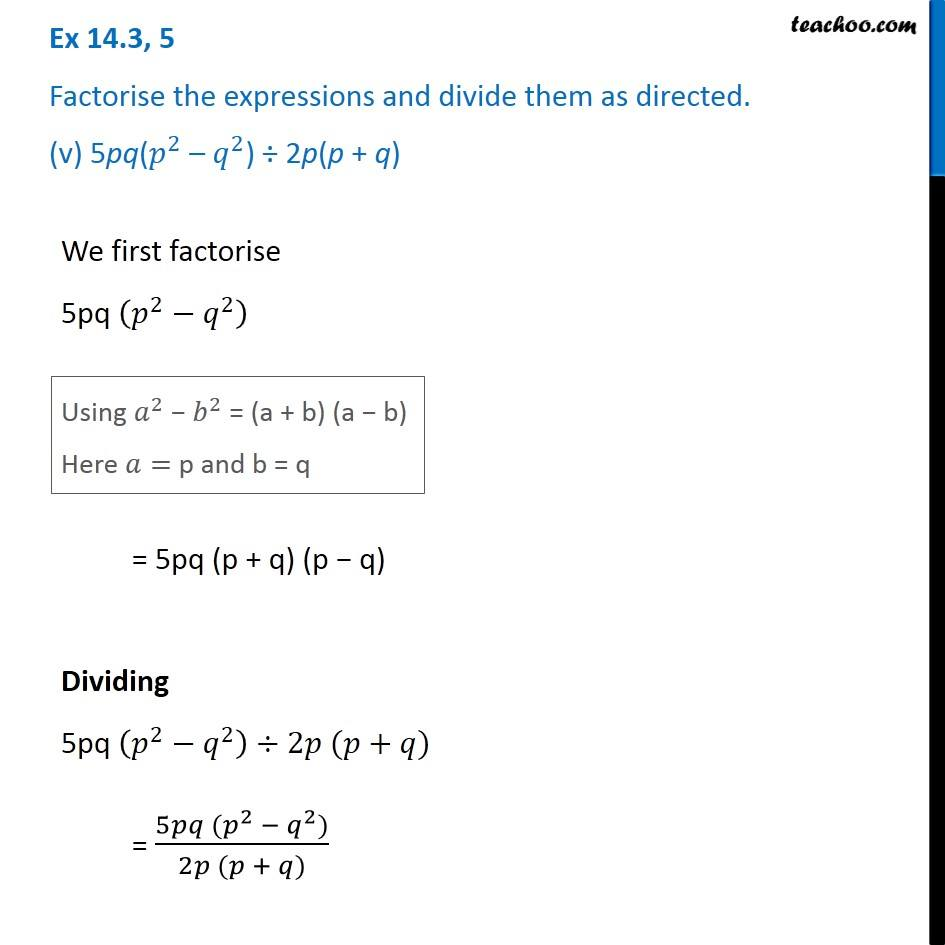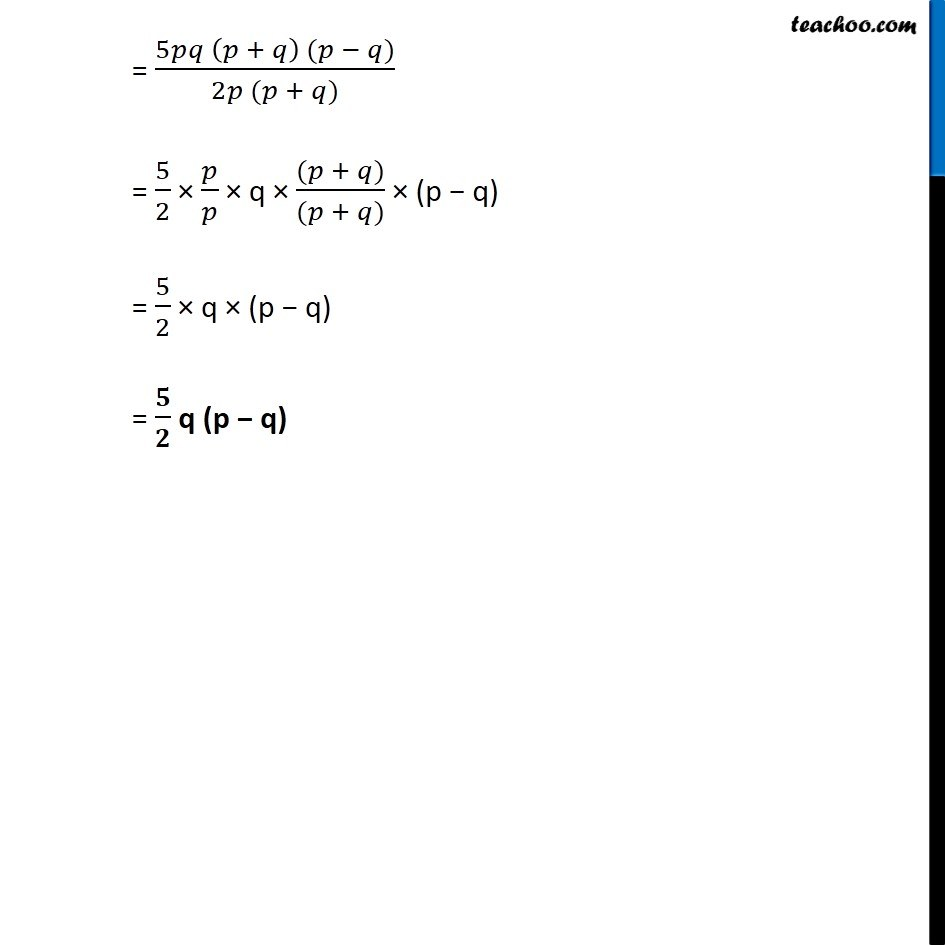1. Chapter 14 Class 8 Factorisation
2. Serial order wise
3. Ex 14.3

Transcript

Ex 14.3, 5 Factorise the expressions and divide them as directed. (v) 5pq(𝑝^2 – 𝑞^2) ÷ 2p(p + q) We first factorise 5pq (𝑝^2−𝑞^2 ) Using 𝑎^2 − 𝑏^2 = (a + b) (a − b) Here 𝑎= p and b = q = 5pq (p + q) (p − q) Dividing 5pq (𝑝^2−𝑞^2 )÷2𝑝 (𝑝+𝑞) = (5𝑝𝑞 (𝑝^2 − 𝑞^2))/(2𝑝 (𝑝 + 𝑞)) = (5𝑝𝑞 (𝑝 + 𝑞) (𝑝 − 𝑞))/(2𝑝 (𝑝 + 𝑞)) = 5/2 × 𝑝/𝑝 × q × ((𝑝 + 𝑞))/((𝑝 + 𝑞)) × (p − q) = 5/2 × q × (p − q) = 𝟓/𝟐 q (p − q)

Ex 14.3